Courses

# Match the following Question of Inverse Trigonometric Functions, Past year Questions JEE Advance JEE Notes | EduRev

## JEE : Match the following Question of Inverse Trigonometric Functions, Past year Questions JEE Advance JEE Notes | EduRev

The document Match the following Question of Inverse Trigonometric Functions, Past year Questions JEE Advance JEE Notes | EduRev is a part of the JEE Course Maths 35 Years JEE Mains & Advance Past year Papers Class 12.
All you need of JEE at this link: JEE

DIRECTIONS (Q. 1 & 2) : Each question contains statements given in two columns, which have to be matched. The statements in Column-I are labelled A, B, C and D, while the statements in Column-II are labelled p, q, r, s and t. Any given statement in Column-I can have correct matching with ONE OR MORE statement(s) in Column-II. The appropriate bubbles corresponding to the answers to these questions have to be darkened as illustrated in the following example :

If the correct matches are A-p, s and t; B-q and r; C-p and q; and D-s then the correct darkening of bubbles will look like the given.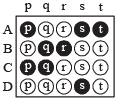Q. 1. Match the following

Column I                                                                                         Column II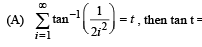(p) 1

(B) Sides a, b, c of a triangle ABC are in AP and                           (q)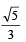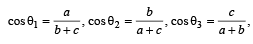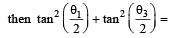(C) A line is perpendicular to x + 2y + 2z = 0 and                        (r) 2/3
passes through (0, 1, 0). The perpendicular
distance of this line from the origin is

Ans. (A)-(p), (B)-(r), (C)-(q)

Solution.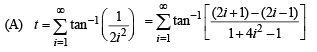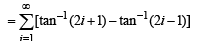t = tan–1 3 – tan–1 1 + tan–15 – tan–13 + ...... + tan–1(2n + 1) – tan–1 (2n – 1) + ...... ∞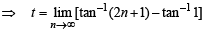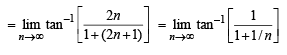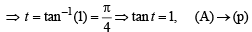(B) ∴ a, b, c are in AP  ⇒ 2b = a + c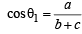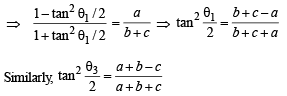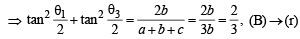(C) Equation of line through (0, 1, 0) and perpendicular to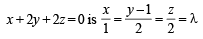For some value of λ, the foot of perpendicular from origin to line is (λ, 2λ + 1, 2λ)
Dr ’s of this ^ from origin are λ , 2λ + 1, 2λ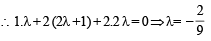∴ Foot of perpendicular is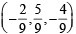∴ Required distance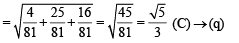Q. 2. Let (x, y) be such that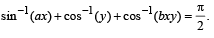Match the statements in Column I with statements in Column II and indicate your answer by darkening the appropriate bubbles in the 4 × 4 matrix given in the ORS.

Column I                                                                    Column II

(A) If a = 1 and b = 0, then (x, y)                                (p) lies on the circle  x2 + y2 = 1

(B) If a = 1 and b = 1, they (x, y)                                (q) lies on (x2 – 1)(y2 – 1) = 0

(C) If a = 1 and b = 2, then (x, y)                                (r) lies on y = x

(D) If a = 2 and b = 2, then (x, y)                                (s) lies on (4x2 – 1) (y2 – 1) = 0

Ans. (A)  → p, (B) → q, (C) → p , (D) → s

Solution.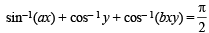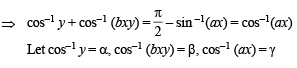⇒ y = cos α, bxy = cos β, αx = cos γ
∴ We get α + β = γ and cos β = bxy
⇒ cos  (γ – α) = bxy
⇒ cos y cos α + sin γ sin α = bxy
⇒ axy + sin γ sin α = bxy ⇒ (a – b) xy = – sin α sin γ
⇒ (a – b)2 x2y2 = – sin2 α sin2 γ
= (1– cos2 α)  (1– cos2 γ)
⇒ (a – b)2 x2y2 = (1– a2x2) (1– y2) ....(1)

(A) For a = 1, b = 0, equation (1) reduces to
x2y2 = (1 – x2) (1– y2) ⇒ x2 + y2 = 1

(B) For a = 1,  b = 1 equation (1) becomes
(1– x2)  (1– y2) = 0  ⇒ (x2 – 1)  (y2 – 1) = 0

(C) For a = 1, b = 2 equation (1) reduces to
x2y2  = (1– x2) (1– y2) ⇒ x2 + y2 = 1

(D) For a = 2,  b = 2 equation (1) reduces to
0 = (1– 4x2)  (1– y2) ⇒ (4x2 – 1)  (y2 – 1)  = 0

DIRECTIONS (Q. 3) : Following question has matching lists. The codes for the lists have choices (a), (b), (c) and (d) out of which ONLY ONE is correct.

Q. 3. Match List I with List II and select the correct answer using the code given below the lists :

List I                                                                                                                                           List II

P.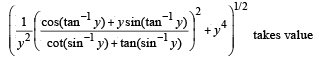1.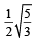Q. If cosx + cosy + cosz = 0 = sinx + siny + sinz then possible value of cos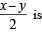2. √2

R.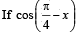cos 2x + sinx sin 2 secx = cosx sin2x secx +                                            3. 1/2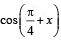cos 2x then possible value of secx is

S.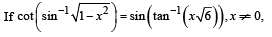4. 1
then possible value of x is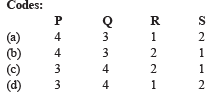Ans. (b)

Solution.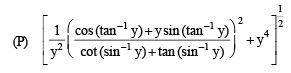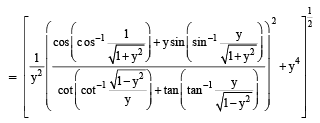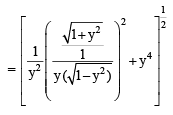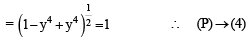(Q) We have cos x + cos y = – cos z
sin x + sin y = – sin z

(cos x + cos y)2 + (sin x + sin y)2 = cos2 z + sin2 z
⇒ 2 + 2 cos (x – y) = 1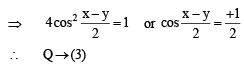(R) We have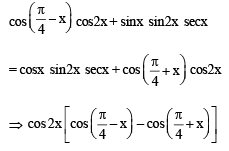= sin2x  secx  (cosx  – sinx)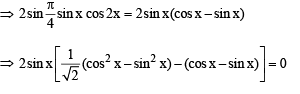∴ (R) → (2)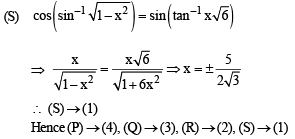Offer running on EduRev: Apply code STAYHOME200 to get INR 200 off on our premium plan EduRev Infinity!

63 docs|43 tests

,

,

,

,

,

,

,

,

,

,

,

,

,

,

,

,

,

,

,

,

,

,

,

,

;